# Three tributaries

It is possible to fill the pool with three tributaries. The first would take 12 hours, the second 15 hours, and the third 20 hours. The day before the summer season began, the manager opened all three tributaries simultaneously. How long did it take to fill the pool?

t =  5 h

### Step-by-step explanation:

t •( 1/12+1/15+1/20) = 1

12t = 60

t = 5

Our simple equation calculator calculates it.Did you find an error or inaccuracy? Feel free to write us. Thank you!Tips to related online calculators
Do you have a linear equation or system of equations and looking for its solution? Or do you have a quadratic equation?
Tip: Our volume units converter will help you with the conversion of volume units.
Do you want to convert time units like minutes to seconds?

## Related math problems and questions:

• Two tributaries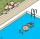Two tributaries of the pool fill it in 10 hours. One of the tributaries would fill 15 hours. How long would fill the first tributary?
• Three pumpsWe are filling the pool. The first pump would be filled in 12 hours, the second pump in 15 hours. If all three pumps were running at the same time, it would fill the pool for 4 hours. How long would the pool fill only with the third pump?
• Tributaries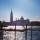The first tributary fill pool with water in 15 hours. The second tributary fill pool in 10 hours. For how many hours the pool is filled with both tributaries?
• Three peopleThree people start doing a work at a same time. The first worked only 2 hours. The second ended 3 hours before the end. On an individual basis, it would take the first time to do the work 10 hours, second 12 hours and 15 hours third. How many hours did it
• Tributaries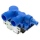We can fill the pool with two different tributaries. The first inflow would fill the pool in 18 hours, both in 6 hours. How many hours would the pool fill with a second inflow?
• Pumps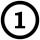First pump flows 16 liters per second into the basin by the second pump 75% of the first and by third pump half more than the second. How long will take fill basin by all three pumps simultaneously volume 15 m3 (cubic meters)?
• Pool 2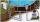The first supply by the pool fill for five hours and the second fill for six hours, drain should be drained for 15 hours. For how many hours the pool is full, when we open both inlet now and outlet open two hours later?
• Pool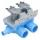Pool is filled with two water supply. The first supply fill pool for nine hours, the second for six hours. How many hours will take fill the pool when the water flows in through the first supply 3 hours and then we will open a second supply?
• Digging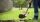The first worker would dig a trench in 6 hours. For the second, the same work would take 4 hours. How long did it take for the trench to be dug if they worked together?
• Digging companies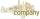Company A would dig a pit for 12 days, company B for 15 days, company C for 20 days and the company D for 24 days. Work began together companies C and D, but after three days joined them other two companies. How long took to dig a pit?
• Three pumps together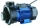One pump fills the tank in 1.5 hours, the second in 2 hours, and the third in 3 hours 20 minutes. How many minutes will the tank fill with three pumps if they work simultaneously?
• Two pumps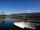The first pump fills the tank in 24 hours second pump in another 40 hours. For how long will it take to fill the tank if it operates both pumps at the same time?
• Bricklayers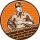The first bricklayer would build a wall in 3 days, the second in 4 days. The first bricklayer worked alone for a day, then the second bricklayer came to his aid and they finished the work together. How long did it take to build the wall?
• Two pipesHow long will the pool be filled with a double supply pipe if it takes the pool to fill the first pipe by 4 hours longer and the second pipe 9 hours longer than both pipes open at the same time?
• Two workers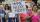The first worker completed the task by himself in 9 hours, the second in 15 hours. After two hours of joint work left first worker to a doctor and the second finished the job himself. How many hours worked second worker himself?
• Oil tank and pipes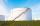The underground oil tank can be filled by two oil pipelines. The first is filled in 72 hours and the second in 48 hours. How many hours from the moment when first pipeline began to fill the oil is it necessary to start filling it with the second to fill i
• Three workers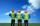Three workers A, B, C have to work on a specific task. Workers A and B completed whole task in 14 days, B together with C for 23 days, A together with C for 13 days. How long it would take to complete the task every one of them alone? How long it would ta